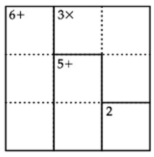Chapter 1.1, Problem 1EEMathematical Excursions (MindTap C...

4th Edition
Richard N. Aufmann + 3 others
ISBN: 9781305965584

Solutions

Chapter
SectionMathematical Excursions (MindTap C...

4th Edition
Richard N. Aufmann + 3 others
ISBN: 9781305965584
Textbook Problem

EXCURSION EXERCISESSolve each of the, following puzzles. Note: The authors of this textbook are not associated with the KenKen brand. Thus the following puzzles are not official KenKen puzzles; however, each puzzle can be solved using the same techniques one would use to solve an Official KenKen puzzle.To determine

The solution of the given puzzle.

Explanation

Given information:

Concept Used:

• As per the kenken rules of puzzles the numbers that can be used for 4×4 puzzles are 1,2,3,and4 . A 6×6 puzzle may use numbers 1 through 6.
• Also each box of the puzzle must contain a required number. If there is more than one box then target is found using the arithmetic operations.

Calculation:

The given puzzle is

Now apply the above mentioned concept.

The above box can be highlighted as below

Still sussing out bartleby?

Check out a sample textbook solution.

See a sample solution

The Solution to Your Study Problems

Bartleby provides explanations to thousands of textbook problems written by our experts, many with advanced degrees!

Get Started

In Exercises 27-30, evaluate each quantity. C(20,18)

Finite Mathematics for the Managerial, Life, and Social Sciences

Evaluate the definite integrals in Problems 1-32. 20.

Mathematical Applications for the Management, Life, and Social Sciences

For and , a × b =

Study Guide for Stewart's Multivariable Calculus, 8th

In Exercises 4756, solve the given equation for the indicated variable. 4=2x

Finite Mathematics and Applied Calculus (MindTap Course List)

For the following scores, find the value of each expression: X 3 2 4 2 a. X b. (X)2 c. X 2 d. (X 2)

Essentials of Statistics for The Behavioral Sciences (MindTap Course List)

Sketch the graph of the set. 37. {(x, y) | x2 + y2 1}

Single Variable Calculus: Early Transcendentals, Volume I